# How to Use the COUNT Function in ExcelClaudia Buckley#### Join the Excel conversation on Slack

Ask a question or join the conversation for all things Excel on our Slack channel.

Excel is all about data - mostly data in large quantities. So it makes sense that at some point, we’ll want to count elements within that data. Luckily, there are functions to help you count in Excel, in the “COUNT” family of functions in the statistical group of the Excel Function Library.

This resource explains how to use the COUNT function in Excel, as well as some helpful related functions in the COUNT family.

## Comparing functions in the COUNT family

To understand what gets counted and what doesn’t when using the different COUNT functions, the following diagram will be useful.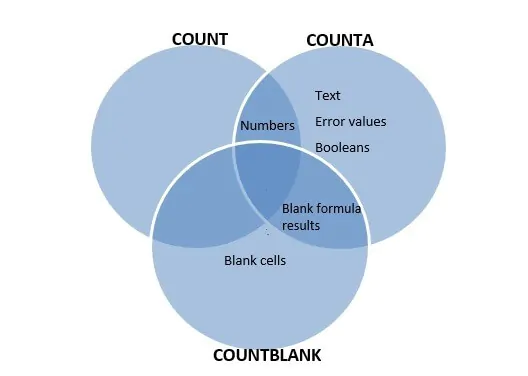The following functions are covered in this resource:

Use this free Excel file to practice along with the COUNT tutorial.

## Using the COUNT function

As with many functions in Excel, the COUNT function is very specific. It counts the number of cells within a range or list that are classified as numbers. The syntax is:

``=COUNT(value1, [value2]...)``

Each value, or argument, within the syntax refers to a cell, range or item in a list. Up to 255 arguments are accepted. The following two examples explain how the COUNT function works.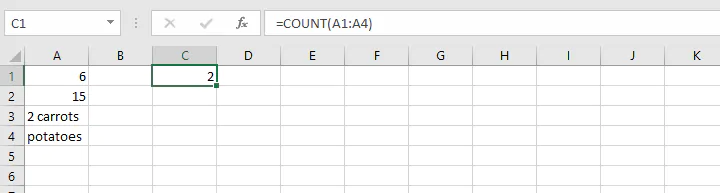The COUNT formula in cell C1 refers to the values in the range A1 to A4, and evaluates them as having two numeric values (6 and 15). The value in cell A4 is obviously text and would not be counted, but A3 is also evaluated as text. The reason for this is that cells which contain both text and numbers are treated as text by Excel. One clue is that Excel automatically aligns numeric values to the right, and text values to the left.

Dates, currencies and percentages and formulas which return a numeric result are also treated as numbers, while error values and Booleans are not. See below for an example of all the above within a range.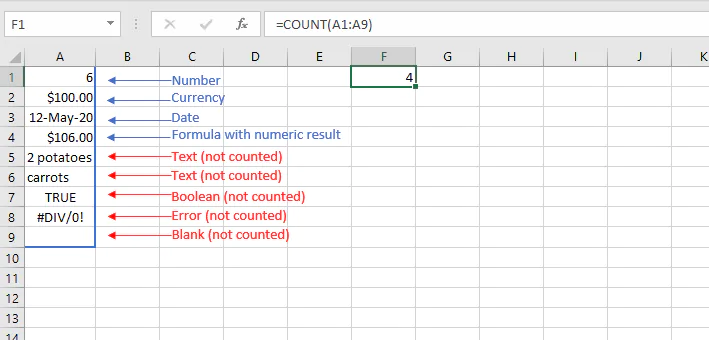The formula =COUNT(A1:A9) in cell F4 evaluates cells A1, A2, A3 and A4 as numbers, while the other cells are not and therefore a result of 4 is returned.

The COUNT function in Excel can also count the number of numeric values if they are listed within the formula itself, so =COUNT(0,4,17) would return a result of 3.

## Count cells with all types of data with COUNTA

On the other hand, if we want to count all cells which contain data - whether text, numbers, formulas, Booleans, or error values - we would use the COUNTA function. The syntax of the COUNTA function is similar to COUNT:

``=COUNTA(value1, [value2]...)``

This formula will count all non-blank cells within a range. The COUNTA function will tell us exactly how many cells have data.

In the example below, COUNTA returns a value of 6 non-empty cells since it counts the text, numbers and error values within the range B2 to B8.

``=COUNTA(B2:B8)``

##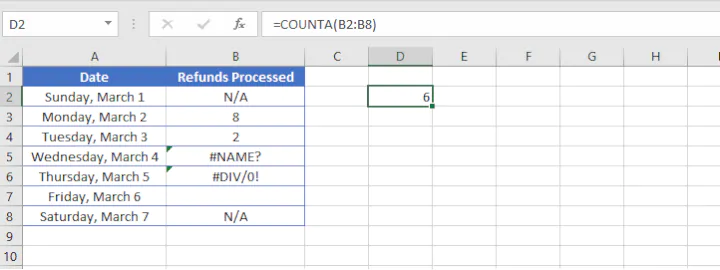Why isn’t my COUNTA formula working?

There may be times when your COUNTA formula gives an unexpected result. It is important to remember that a cell which appears to be empty may, in fact, contain a space or a formula which gives it an appearance of being empty. The COUNTA function will include these cells in its non-empty cell count also.

This is shown in the following example. The same formula, =COUNTA(B2:B8), is entered in cell D2, returning a result of 6, even though cells B5 and B6 appear to be empty.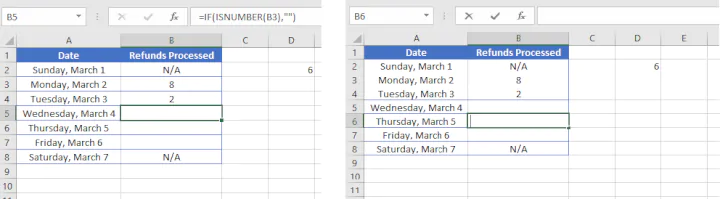Cell B5 above contains a formula which returns a blank cell, and cell B6 contains a space, which is a text value. Therefore, only cell B7 was excluded from the COUNTA tally, bringing the total to 6 cells with data. Learn how to eliminate this problem here.

## Count blank cells

There are other times when we want to know the opposite - how many cells are blank. Blank cells hint at missing or incomplete data, such as in the example below. The COUNTBLANK function is perfect for telling us how many cells have nothing in them.

The syntax is similar to the COUNT and COUNTA functions:

``=COUNTBLANK(range)``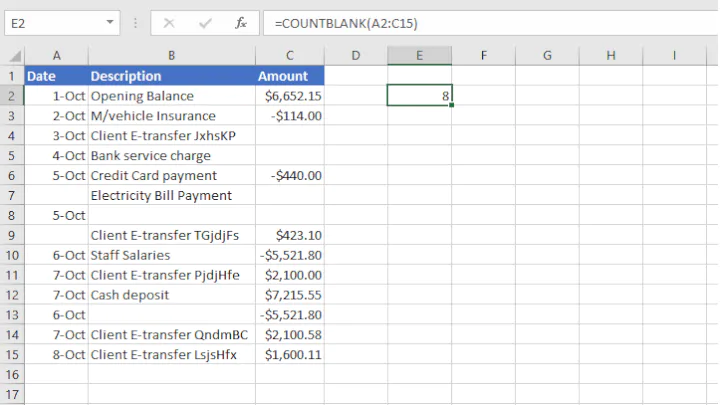The COUNTBLANK formula in cell E2 checks the range A2 to C15 and determines that eight cells contain no data.

It is worth remembering that if a cell contains a formula which returns a blank value, the COUNTBLANK function also counts this cell as a blank.

## Count the number of cells within a range

There may also be times when you just want to know the total number of cells in a range. While there is not one specific function for that, fortunately, there is a workaround. We can multiply the number of rows in an array by the number of columns with the format:

``=ROWS(array)`*`COLUMNS(array)``

##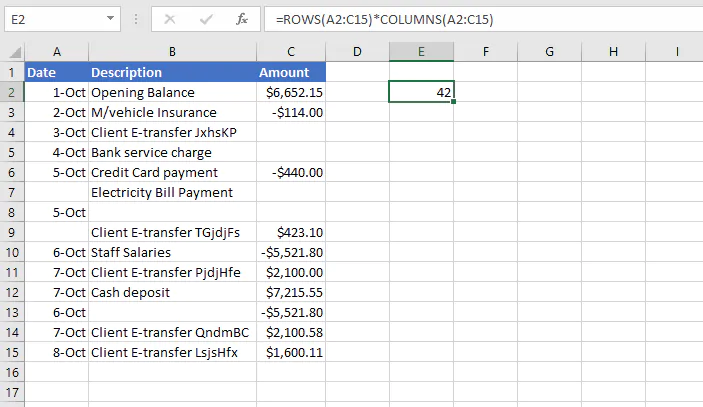Count cells which satisfy certain conditions

### Count cells using a single condition

There are also functions within the “COUNT” family which can count how many times a specific value or text appears within a range. The COUNTIF and COUNTIFS functions allow you to specify the criteria which Excel will use to evaluate the range of cells.

The COUNTIF syntax is:

``=COUNTIF(range, criteria)``

For example, the formula below counts the number of cells which contain the text “e-transfer”.

``=COUNTIF(A2:C15, `“*e-transfer*”`)``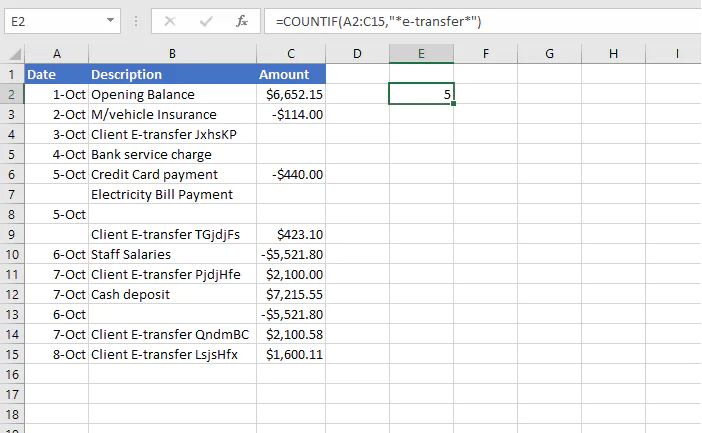Note that an asterisk wildcard is placed before and after the word “e-transfer” so as to include all occurrences where “e-transfer” is a part of a text string within the specified range.

### Count cells using multiple conditions

The COUNTIFS function counts the number of cells which satisfy all the conditions stated within the formula.

The COUNTIFS syntax is:

``=COUNTIFS(criteria_range1, criteria1, [criteria_range2, criteria2],...)``

For example, the formula below counts the number of cells within the range C2 to C15 which are greater than 0 and less than 2000.

``=COUNTIFS(C2:C15,">0",C2:C15,"<2000")``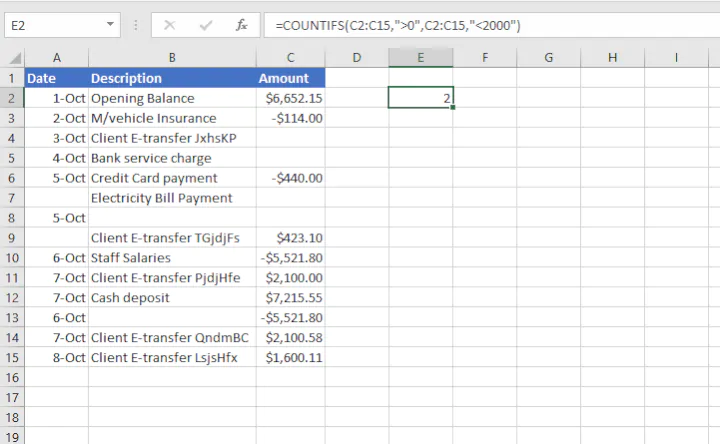Only the values in cells C9 and C15 satisfy both criteria, so a value of 2 is returned.

The COUNTIF function can count the number of cells which satisfy a single condition, while the COUNTIFS function allows up to 127 criteria.

## Count the number of characters in a string

Something else we might want to count is the number of characters within a string. The LEN function counts the length of a string, whether that string appears within a cell or is typed directly into the formula.

Take for example, the formula =LEN(B3) in the spreadsheet below.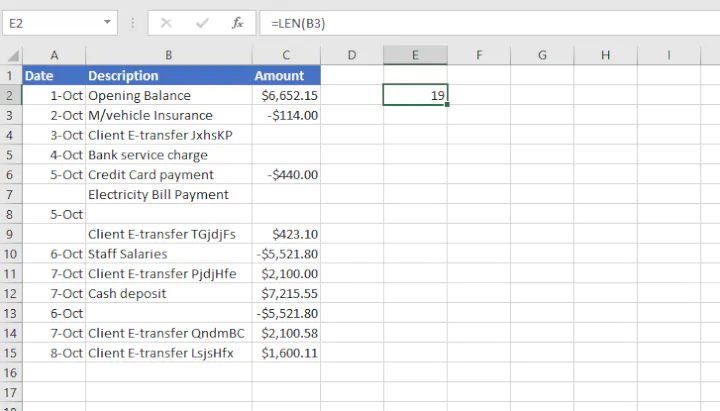This formula counts the number of letters, spaces and special characters in the string “M/vehicle Insurance” and returns a value of 19.

The result would have been the same if we had entered:

``=LEN("M/vehicle Insurance")``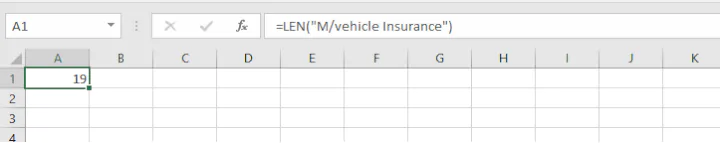Even though LEN is classified as a text function in the Excel Function Library, we could consider it a “counting” function, since it also counts the length of characters in a numerical set.

Here’s an interesting example. The LEN function counts the number of characters in cell C2 below, returning a value of 7.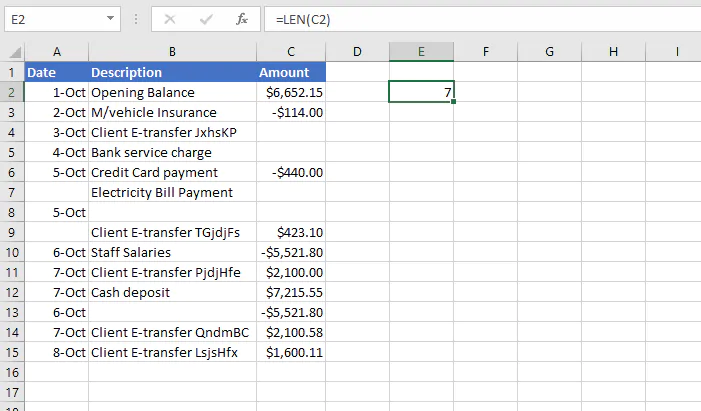Although it seems that there are 9 characters in cell C2, the dollar sign and comma were simply displayed because of the number format applied to that cell. The true characters (6652.15) are shown in the Formula Bar (see below):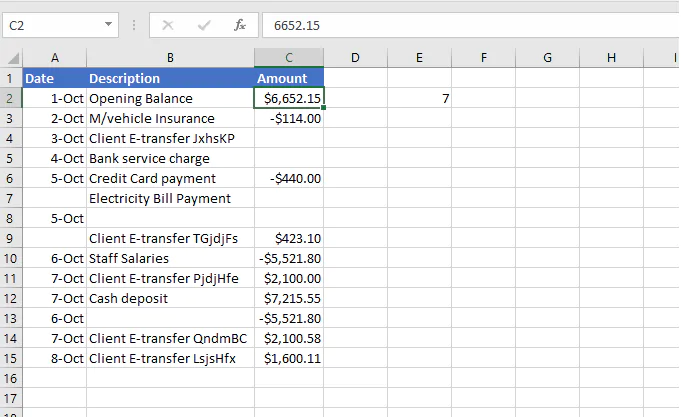## Ready to put the COUNT function to use?

So, what would you like to use the COUNT function for? Excel is ready to help, and GoSkills is ready to help you learn how.

Check out our Microsoft Excel - Basic and Advanced course to master more formulas and functions that you can put to use and begin enhancing your productivity today!

Become a certified Excel ninja with GoSkills bite-sized courses#### Join the Excel conversation on Slack

Ask a question or join the conversation for all things Excel on our Slack channel.Claudia Buckley

Claudia is a project manager and business skills instructor at GoSkills. In her spare time, she reads mystery novels and does genealogy research.